CBSE Sample Papers for Class 12 Economics Paper 4 are part of CBSE Sample Papers for Class 12 Economics. Here we have given CBSE Sample Papers for Class 12 Economics Paper 4.

## CBSE Sample Papers for Class 12 Economics Paper 4

 Board CBSE Class XII Subject Economics Sample Paper Set Paper 4 Category CBSE Sample Papers

Students who are going to appear for CBSE Class 12 Examinations are advised to practice the CBSE sample papers given here which is designed as per the latest Syllabus and marking scheme as prescribed by the CBSE is given here. Paper 4 of Solved CBSE Sample Paper for Class 12 Economics is given below with free PDF download solutions.

Time : 3 hrs
M.M.: 80

General Instructions

• All questions in both the sections A and B are compulsory. However, there is internal choice in questions of 3,4 and 6 marks.
• Question Nos. 1-4 and 13-16 are very short answer type questions, carrying 1 mark each.
They are required to be answered in one sentence each.
• Question Nos. 5-6 and 17-18 are short answer I type questions, carrying 3 marks each.
Answers to them should not normally exceed 60 words each.
• Question Nos. 7-9 and 19-21 are short answer II type questions, carrying 4 marks each.
Answers to them should not normally exceed 70 words each.
• Question Nos. 10-12 and 22-24 are long answer type questions, carrying 6 marks each.
Answers to them should not normally exceed 100 words each.
• Answers should be brief and to the point and the above word limit be adhered to as far as possible.

Section – A

Question 1.
State the economic value achieved through the spread of education in the context of production potential.

Question 2.
Why is fixed cost curve parallel to X-axis?

Question 3.
The application of fixed factor can be changed during
(a) long period
(b) short period
(c) short as well as long period
(d) None of these

Question 4.
A businessman invests his savings in a business and employs professionals to manage business. Identify implicit cost here.

Question 5.
Find out Marginal Opportunity Cost (MOC) of good X in the following schedule and also comment on the probable shape of the production possibility curve.

 Production of Good X (units) Production of Good Y (units) 0 15 1 14 2 12 3 9 4 5 5 0

Question 6.
Differentiate between normal goods and inferior goods?
Or
What do you understand by the rightward shift in the demand curve? Explain it with the help of an example and diagram.

Question 7.
What do you understand by price ceiling? Write its two major implications.
Or
Explain the impact on equilibrium price and quantity when
(i) Demand is perfectly elastic and supply falls.
(ii) Supply is perfectly inelastic and demand rises.

Question 8.
A consumer consumes only two goods X and Y both priced at ₹ 8 per unit. If the consumer chooses a combination of these two goods with marginal rate of substitution equal to 8, is the consumer is equilibrium? Give reasons. Also explain, what will a rational consumer do in this situation?

Question 9.
Draw Average Revenue (AR) and Marginal Revenue (MR) curves in a single diagram of a firm which can sell more units of a good only by lowering the price of that good. Explain.

Question 10.
Is a producer in equilibrium under the following situations?
(i) When Marginal Revenue (MR) is greater than Marginal Cost (MC).
(ii) When Marginal Revenue (MR) is equal to Marginal Cost (MC).

Question 11.
Explain the different conditions in which a budget line can change. Ramjas always tries to buy that basket of goods which lies on his budget line. Identify the economic value.

Question 12.
What do you understand by product differentiation? Also, explain the implications of feature product differentiation in monopolistic market.
Or
At a given price of a commodity there is ‘excess supply’. Is this the equilibrium price? If not, how will the equilibrium price be reached?

Section – B

Question 13.
What is meant by fiscal discipline?

Question 14.
Write one implication of revenue deficit of the government.

Question 15.
Which of the following systems is followed by Reserve Bank of India for issuing currency?
(a) Minimum reserve system
(b) Proportionate system
(c) Fixed fiduciary issue system
(d) Simple deposit system

Question 16.
Explain the meaning of credit money.

Question 17.
What will happen to an economy if aggregate demand falls below full employment level? Explain using a graph.
Or
Explain the role of margin requirement in correcting the problem of deflationary gap.

Question 18.
In an economy, the equilibrium level of income is ₹ 12,000 crore. The ratio of Marginal Propensity to Consume (MPC) and Marginal Propensity to Save (MPS) is 3:1. Calculate the additional investment needed to reach a new equilibrium level of income of ₹ 20,000 crore. Identify the economic value involved in increasing the investment in the economy.

Question 19.
Explain the circular flow of income in a two sector economy with the help of a diagram.

Question 20.
What is bank rate? How does it work as a method of credit control?
Or
“Introduction of money has separated the acts of sale and purchase”, Explain how?

Question 21.
Explain the importance of government budget.

Question 22.
Giving reasons, explain the treatment assigned to the following while estimating national income.
(i) Expenditure on adding a floor to building.
(ii) Payment of fees to a lawyer engaged by a firm.
(iii) Payment of interest by a government firm.
Or
Calculate National Income by
(i) Product method
(ii) Expenditure method.

 S.No. Items (₹) in crores (i) Gross Value Added at Market Price by Primary Sector 600 (ii) Private Final Consumption Expenditure 1,500 (iii) Consumption of Fixed Capital 300 (iv) Net Indirect Taxes 240 (V) Gross Value Added at Market Price by Secondary Sector 400 (Vi) Net Domestic Fixed Capital Formation 440 (vii) Change in Stock H40 (viii) Gross Value Added at Market Price by Tertiary Sector 1,400 (ix) Net Imports 100 (x) Government Final Consumption Expenditure 300 (xi) Net Factor Income from Abroad 40

Question 23.
Distinguish between inflationary gap and deflationary gap. Name two measures by which these can be corrected.

Question 24.
(i) How is fixed rate of exchange determined?
(ii) Explain the main merits of flexible exchange rate.

Government’s endeavours to spread education will lead to an increase in the quality of workforce. The production potential of the country would also increase. Economic value reflected is in terms of providing better quality of workforce. Also, this is expected to shift the PPC rightwards.

Fixed cost remains constant at all levels of output. So, fixed cost curve is parallel to X-axis.

(a) The application of fixed factor can be changed during long period.

Loss of interest on his savings is the implicit cost of businessman.

 Production of Good X (units) Production of Good Y (units) Marginal Opportunity Cost (MOC) 0 15- – 1 14. $$\frac { 1 }{ 1 } =1$$ 2 12 $$\frac { 2 }{ 1 } =2$$ 3 9 $$\frac { 3 }{ 1 } =3$$ 4 5 $$\frac { 4 }{ 1 } =4$$ 5 0 $$\frac { 5 }{ 1 } =5$$

Marginal Opportunity Cost $$\left( MOC \right) =\frac { Loss\quad of\quad Output\quad of\quad Y\left( \triangle Y \right) }{ Gain\quad of\quad Output\quad of\quad X\left( \triangle X \right) }$$
Since, MOC is rising, therefore Production Possibility Curve (PPC) will be concave to origin.

Difference between normal goods and inferior goods are:

 Basis Normal Goods Inferior Goods Income effect Income effect is positive. Income effect is negative. Essential to life Normal goods may or may not be essential to life. Inferior goods are generally essential to life due to which only they are demanded even being inferior. Law of demand These goods obey law of demand. These goods may or may not obey law of demand.

Or
Due to changes in factors other than the price of the commodity concerned, if more quantity at the same price is demanded, then it is termed as increase in demand and is represented by the rightward shift in the demand curve.
Increase in demand can be explained better with the below mentioned example and diagram:

 Price (₹) Quantity Demanded (units) 10 10 10 25

As it is clear from the above schedule that at the same price of ₹ 10, demand has increased from 20 to 25 units.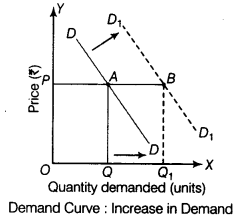In the given diagram, demand increases from OQ to OQ1 at the same price OP.

Price ceiling refers to the maximum price of a commodity that the sellers can charge from the buyers. It is generally imposed on essential commodities like foodgrains and certain medicines.Implications
Government fixes this price lower than the equilibrium market price, so that the concerned commodity remains within the reach of poorer sections of society. Due to this, following implications of price ceiling are seen:

1. Since ceiling price is lower than the equilibrium price, there is likely to be excess demand in the market, leading to shortage of commodity.
2. To make extra normal profits, sellers generally resort to black marketing.

Or
(i) When demand is perfectly elastic and supply decreases, there will be no change in price but the equilibrium quantity will decrease. The following diagram shows that when supply decreases from SS to S1S1, equilibrium price remains constant at OP but equilibrium quantity decreases from OQ to OQ1.(ii) When supply is perfectly inelastic and demand increases, the price of the commodity will increase but the equilibrium quantity of that commodity remains constant. The following diagram shows that when demand increases from DD to D1D1, the equilibrium price also increases from OP to OP1 but equilibrium quantity of that commodity remains constant at OQ.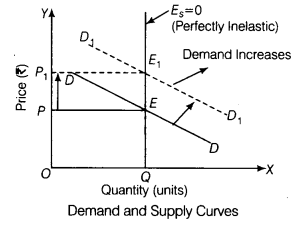Given, PX ₹8 PY ₹8 MRSXY = 8
Consumer’s equilibrium is attained where
$${ MRS }_{ XY }=\frac { { P }_{ X } }{ { P }_{ Y } } \Rightarrow 8>\frac { 8 }{ 8 } ;$$
∴ $${ MRS }_{ XY }>\frac { { P }_{ X } }{ { P }_{ Y } }$$, so the consumer is not in equilibrium.
Here, consumer can attain equilibrium only when MRSXY starts falling and becomes equal to $$\frac { { P }_{ X } }{ { P }_{ Y } }$$ (on the assumption that $$\frac { { P }_{ X } }{ { P }_{ Y } }$$ is constant). This happens only when consumer starts consuming more of X in place of Y so, that he moves downward to right along the IC.
So, if $${ MRS }_{ XY }=\frac { { P }_{ X } }{ { P }_{ Y } }$$, then a rational consumer would react by substituting X for Y.

A firm can sell more only by lowering the price in imperfect market forms such as monopoly and monopolistic competition. In these market forms, the Average Revenue (AR) curve is downward sloping. When AR curve is falling, Marginal Revenue (MR) is less than AR. Therefore, MR curve lies below the AR curve. The slope of MR is half the slope of AR.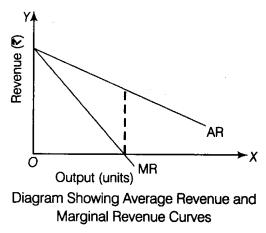It can be seen from the above diagram that at the mid-point of AR curve, MR is zero and below mid-point of AR, MR becomes negative.

A producer is said to be in equilibrium when he is earning maximum profits and he has no tendency to change the level of output. Producer’s equilibrium under the following situations :

1. When Marginal Revenue is Greater than Marginal Cost (MR > MC) Under this situation, the producer will not be in equilibrium because at this point, the marginal revenues from the sale of an additional unit exceeds the marginal cost incurred in producing that good.
As a result, it will be more profitable for the producer to expand the level of output till MR becomes equal to MC and MC exceeds MR beyond the point of equality.
2. When Marginal Revenue is Equal to Marginal Cost (MR = MC) Under this situation, the producer or
firm may or may not be in equilibrium because :
At this point of equality of MR and MC, if MC exceeds MR beyond the point of equality, only then the producer will be in equilibrium because MR = MC is necessary but not a sufficient condition of producers’ equilibrium. The producer will be in equilibrium only when following two conditions are satisfied simultaneously i.e.,
MR = MC and MC must cut MR from below.

The budget line of a consumer can change in the conditions discussed below:
(i) When Income of the Consumer Changes Assuming price to be constant, budget line will change with a change in consumer’s income in the following ways :
(a) When Income of the Consumer Increases When income of the consumer increases, with no change in the prices of good X and good Y, then there will be a parallel rightward shift in the budget line.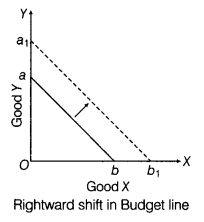With increase in consumer’s income, budget line shifts from ab to a1b1.
(b) When Income of the Consumer Decreases When income of the consumer decreases, with no change in the price of good X and good Y, then there will be a parallel leftward shift in the budget line.With decrease in consumer’s income, budget line shifts from ab to a1b1.
(ii) When Price of Good X Changes When price of good X changes and price of good Y and income of the consumer remains unchanged, then,
(a) increase in price of good X will lead to consumer buying less units of good X. The budget line will rotate leftwards from ab to ab1.
(b) decrease in price of good X will lead to consumer buying more units of good X. The budget line will rotate rightwards from ab to ab2Budget line rotates inwards when price of good X rises and rotates outwards when price of good X falls.
(iii) When Price of Good Y Changes When price of good Y changes and price of good X and income of the consumer remains unchanged, then,
(a) increase in price of good Y will lead to consumer buying less units of good Y.
The budget line will rotate leftwards from ab to a1b.
(b) decrease in price of good Y will lead to consumer buying more units of good Y.
The budget line will rotate rightwards from ab to a2b.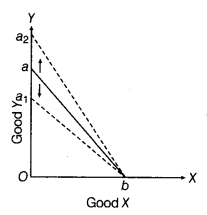Budget line rotates inwards when price of good Y rises and rotates outwards when price of good Y falls,
(iv) When Price of Both the Goods Increases Assuming consumer’s income to be constant, when price of both the goods increase, then budget line will shift leftwards from ab to a1b1.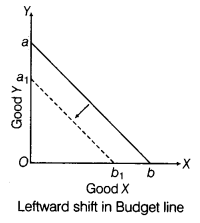Budget line shifts leftwards with increase in the prices of both the goods.
(v) When Prices of both the Goods Decreases Assuming consumer’s income to be constant, when price of both the goods decreases, then budget line will shift rightwards from ab to a1b1.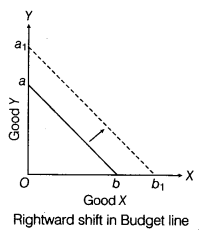Budget line shifts rightwards with decrease in the prices of both the goods.
The economic value is to consume within one’s means.

Product differentiation refers to the practice of differentiating products on lines of design, colour or
packaging. In monopolistic competition, rival firms sell products which are not perfect substitutes but close substitutes of each other e.g. Pepsodent and Colgate toothpaste are close substitutes of each other due to product differentiation in their ingredients.
The implications of monopolistic competition are as follows:

1. It allows firm to have a partial control over price of their product.
2. Elasticity of demand for a product tends to be high due to presence of close substitutes.
3. Consumer’s welfare is increased due to large variety of commodity.

Or
Equilibrium price refers to the price at which market demand is equal to market supply (i.e. there is no excess demand or excess supply). So, the price with ‘excess supply’ is not the equillibrium price.
This can be illustrated with the help of the following figure :The given figure depicts, that excess supply is equal to AB=Q1Q2. It implies that market supply is greater than market demand.
This puts pressure on price (OP1) to decline. The producers reduce the quantity supplied at the lower price OP from OQ2 to OQ. The consumers react by increasing the quantity demanded from OQ1 (at OP1 price) to OQ (at OP price). Equilibrium is struck at point ‘E’. Thus, OP and OQ are the equilibrium price and equilibrium quantity respectively with no excess supply.

Section – B

Fiscal discipline refers to a situation when fiscal deficit is within the manageable limits and it does not lead to high rate of inflation in the country.

Revenue deficit indicates that government is unable to cover its day-to-day expenses which hampers the future growth.

(a) Minimum reserve system is followed by Reserve Bank of India for issuing currency.

Credit money refers to deposits with banks which are withdrawable through a cheque.

If aggregate demand falls below full employment level, it will lead to deflationary situations in the economy.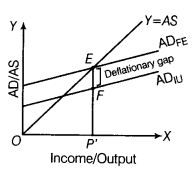ADIU = AD at involuntary unemployment level or below full employment level.
The point E is the equilibrium point, where AD = AS. But at the current levels, deficient demand situation (due to involuntary unemployment) of ADIU, the Aggregate Demand is less than the actual supply in the economy. Hence, EF is the deflationary gap.
Deflationary Gap = Deficient Demand
Or
Margin requirement refers to the margin between the market value of the security provided by the borrower and the loan amount approved. When margin is lower, the borrowing capacity of the borrower is higher.

The Central Bank has the power to change this margin. When Central Bank lowers the margin, the borrowing capacity of the borrower increases. This raises consumption expenditure and Aggregate Demand (AD) also increases which helps in correcting deflationary gap.

Given, $$\frac { MPC }{ MPS } =\frac { 3 }{ 1 }$$
Let MPC be 3x and MPS be 1x
Also, MPC + MPS=1
∴ 3x + 1x = 1 ⇒ 4x = 1
$$x=\frac { 1 }{ 4 } =025$$
Or MPS = x = 025
MPC = 3x = 3 x 025 = 0.75
Now, change in income (∆Y) = ₹ 8,000
$$K=\frac { 1 }{ 1-MPC } =\frac { 1 }{ 1-0.75 } =4$$
$$K=\frac { \triangle Y }{ \triangle / }$$ ⇒ ∆Y = ∆/.K
8,000 = ∆/x 4 ⇒ ∆/ = ₹ 2,000
So, investment in the economy should rise by ₹ 2,000.
The economic value reflected is concern for increasing the productive capacity of the country.

In a two sector economy, there are only two sectors of economic activity namely households and firms. Households supply factor services to firms and firms hire factor services from households. Households spend their entire income on consumption. Firms sell what is produced to the households.
There are two types of markets in this economy:
(i) Product Market Market for goods and services.
(ii) Factor Market Market for factors of production.The above figure shows that

1. Total Production of Goods and Services by firms
= Total Consumption of Goods and Services by Households
2. Factor Payments by Firms = Factor Incomes of Households
3. Consumption Expenditure of Households = Income of Firms
4. Thus, Real Flow of Production and Consumption of Firms and Households = Money Flows of Income
and Expenditure of Firms and Households

Bank rate refers to the minimum rate at which the Central Bank of a country giveS credit to the commercial banks without any collateral. Increase in the bank rate increases the rate of interest and the cost of borrowings goes up, which discourages borrowers from taking loans which reduces the ability of commercial banks to create credit. On the other hand, a decrease in the bank rate decreases the rate of interest and the cost of borrowings goes down, which encourages borrowers to demand more loans and credit is expanded.
Or
Under the Barter system of exchange, acts of sale and purchase of a good or service were to occur at the same point of time. To buy a thing, an individual must at the same time sell something needed by the other person. But with the introduction of money (as a medium of exchange), an individual can buy a good with money without selling anything at the same time. Likewise, he can sell a good for money without buying anything at the same time. Thus, with the introduction of money, acts of sale and purchase have been separated.

Importance of government budget can be explained as follows:

1. It shows revenue deficit, fiscal deficit and primary deficit of the government budget which reflects the status of fiscal discipline in the country.
2. It reflects how the government is going to influence the allocation of resources in the country.
3. It points to the efforts of the government to achieve equality in the distribution of income and wealth.
4. It is used to correct the situations of inflationary/deflationary gap in the economy.

Treatment assigned to the following while estimating national income are as follows:

1. Expenditure on adding a floor to building is included in national income because it is a part of investment expenditure.
2. Payment of fees to a lawyer engaged by a firm is not to be included in estimation of national income as it is treated as part of intermediate consumption.
3. Payment of interest by a government firm should be included while estimating national*income because it
is a kind of factor payment.

Or
(i) By Product Method
Gross Domestic Product at Market Price (GDPMP)
= Gross Value Added at Market Price in Primary Sector + Gross Value Added at Market Price in Secondary Sector + Gross Value Added at Market Price in Tertiary Sector
= 600 + 400 + 1,400
GDPMP = ₹ 2,400 crore
Net National Product at Factor Cost (NNPfc) = GDPMP + Net Factor Income from Abroad – Consumption of . Fixed Capital – Net Indirect Tax
=₹ 2,400 + 40 – 300 – 240 ⇒ ₹ 2,440 – 300 – 240
∴ NNPFC = ₹ 1,900 crore

(ii) By Expenditure Method
Gross Domestic Product at Market Price (GDPMP)
= Private Final Consumption Expenditure
+ Government Final Consumption Expenditure
+ Gross Domestic Capital Formation (Net Domestic Fixed Capital Formation + Consumption of Fixed Capital + Change in Stock) + Net Exports
= ₹ 1,500 + 300 + [440 + 300 + (-40)] + (-100)
= ₹ 1,800 + 700 -100 ⇒ ₹ 2,500 -100
∴ GDPMP = ₹ 2,400 crore
Net National Product at Factor Cost (NNPFC)
= GDPMP – Net Indirect Tax + Net Factor Income from Abroad – Depreciation
= ₹ 2,400 – 240 + 40 – 300 ⇒ ₹ 2,440 – 540
NNPFC = ₹ 1,900 crore

Difference between inflationary gap and deflationary gap are :

 Basis Inflationary Gap Deflationary Gap Meaning The excess of aggregate demand above the level that is required to maintain full employment level of equilibrium is termed as inflationary gap. The short fall of aggregate demand below the level that is required to maintain full employment level of equilibrium is termed as ‘ deflationary gap. Effect on price level Inflationary gap causes inflation and increases wages and price level in the economy. Deflationary gap causes deflation and decreases wages and price level in the economy. Level of output Level of output is constant at full employment. Level of output is less than that at full employment. Level of employment There is no unemployment or underemployment in the economy. There is unemployment or underemployment in the economy.

Two measures by which inflationary gap can be corrected:

1. Increase in bank rate
2. Increase in repo rate

Two measures by which deflationary gap can be corrected:

1. Decrease in bank rate
2. Decrease in repo rate

Then, 1 \$ = $$\frac { 20 }{ 5 }$$ = ₹ 4